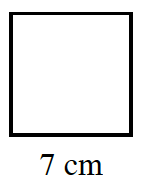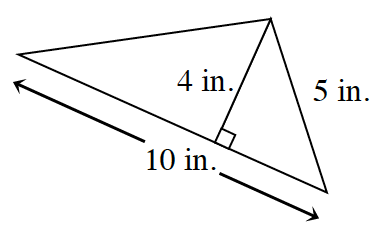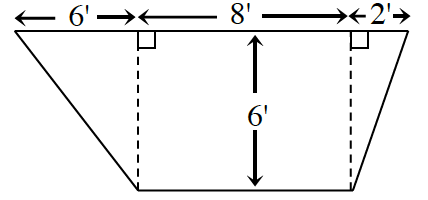Home > CCG > Chapter 2 > Lesson 2.2.4 > Problem2-94

2-94.

Find the area of each figure below. Show all work. Remember to include units in your answer. Homework Help ✎

Look at the Math Notes box in Lesson 2.2.4 for help on how to find the areas of different shapes.

1. a square:$49$ square cm

2.3.$\frac{1}{2}(b_{1}+b{_{2}})h$

$\frac{1}{2}(16+8)6\text{ feet}^2$